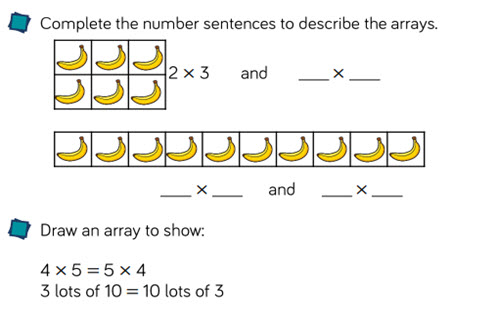# Multiplication and division: multiply and divide by 3, 4 and 8

This guide provides a sequence of small steps starting with a recap of prerequisite understandings. Explore multiplication concepts of equal groupings before a focus on multiplying and dividing by 3, 4 and 8.

Year level(s) Year 2, Year 3, Year 4
Audience Teacher
Purpose Teaching resource, Teaching strategies
Teaching strategies and pedagogical approaches Questioning, Concrete Representational Abstract model, Explicit teaching
Keywords repeated addition, arrays, times tables, skip-counting, sharing, grouping, bar model, explicit teaching

## Curriculum alignment

Curriculum connections Numeracy
Strand and focus Number, Build understanding
Topics Multiplication and division, Place value
AC: Mathematics (V9.0) content descriptions
AC9M2N05
Multiply and divide by one-digit numbers using repeated addition, equal grouping, arrays, and partitioning to support a variety of calculation strategies

AC9M3A03
Recall and demonstrate proficiency with multiplication facts for 3, 4, 5 and 10; extend and apply facts to develop the related division facts

AC9M3N04
Multiply and divide one- and two-digit numbers, representing problems using number sentences, diagrams and arrays, and using a variety of calculation strategies

AC9M4A02
Recall and demonstrate proficiency with multiplication facts up to 10 x 10 and related division facts; extend and apply facts to develop efficient mental strategies for computation with larger numbers without a calculator

AC9M4N06
Develop efficient strategies and use appropriate digital tools for solving problems involving addition and subtraction, and multiplication and division where there is no remainder

Multiplicative strategies (P5, P6, P7)
Number patterns and algebraic thinking (P4)
Number and place value (P7)